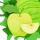Home
IT Knowledge
Inspiration
Languages
EN

# C# / .NET - replace first character in string (range operator ..)

0 points
Created by:Zeeshan-Peel
430

In this article, we would like to show you how to replace the first character in a string in C# / .NET.

Note: range operator `..` was introduced in C# 8.0.

Quick solution:

``````string text = "ABC";
string replacement = "x";
string result = replacement + text[1..];

Console.WriteLine(result);  // xBC``````

## 1. Practical examples

### 1.1 With `+` and range operator

In this example, we will take the `text` string without the first character and add it at the end of the replacement.

``````using System;

public class StringUtils
{
public static void Main(string[] args)
{
string text = "ABC";
string replacement = "x";
string result = replacement + text[1..];

Console.WriteLine(result);  // xBC
}
}``````

### 1.2 With `string.Concat()` method and range operator

This approach is equivalent to the above one. Instead of `+` operator we concatenate the strings using `string.Concat()` method.

``````using System;

public class StringUtils
{
public static void Main(string[] args)
{
string text = "ABC";
string replacement = "x";
string result = string.Concat(replacement, text[1..]);

Console.WriteLine(result);  // xBC
}
}``````

## 2. Practical example using string `Regex.Replace()` with regex pattern

In this example, we use `Regex.Replace()` with `"^."` regex to replace the first character in the `text` string.

Regex explanation:

• `^` - matches the beginning of the string,
• `.` - matches any character except linebreaks.
``````using System;
using System.Text.RegularExpressions;

public class StringUtils
{
public static void Main(string[] args)
{
string text = "ABC";
string replacement = "x";
string pattern = @"^.";
string result = Regex.Replace(text, pattern, replacement);

Console.WriteLine(result);  // xBC
}
}``````